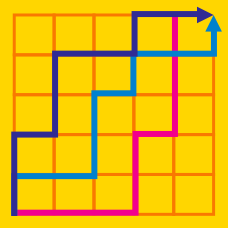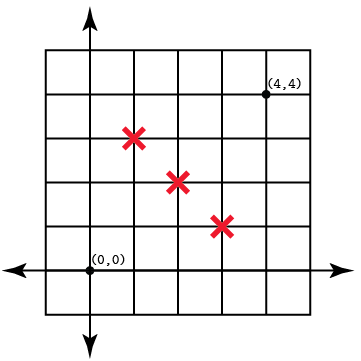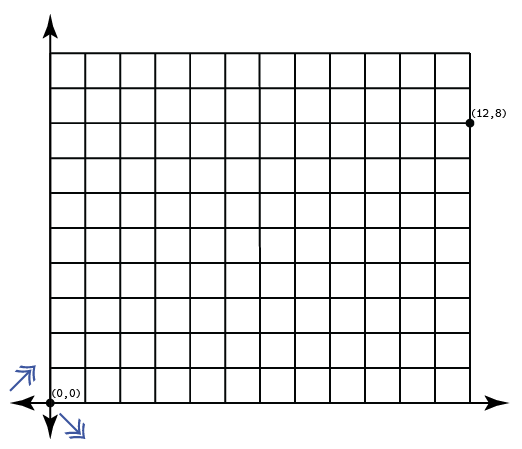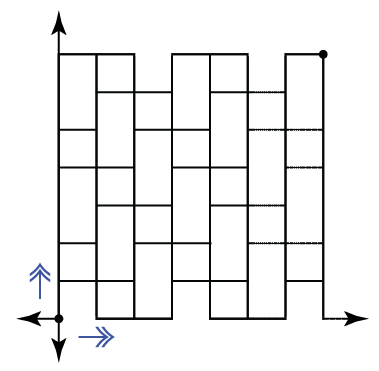Probability

# Rectangular Grid Walk - Problem Solving

An ant in the coordinate plane needs to travel from the origin to $(4, \, 4)$ by moving in $1$ unit increments up or to the right. However, the ant cannot travel through $(3, \, 1)$, $(2, \, 2)$, or $(1, \, 3)$. How many possible paths can the ant take?Wonder Woman needs to rescue a man from a burning building located at $(12, \, 8)$. If she is flying from the origin in diagonal increments of $(+1, \, +1)$ and $(+1, \, -1)$, in how many ways can she reach the burning building?The Three Musketeers are located at the origin and trying to reach d'Artagnan, who is located at $(7, \, 7)$. Each step, they may only move up or right $1$ unit, and every third step, when the sum of coordinates is divisible by $3$, they cannot move to the right. How many paths can they take to d'Artagnan?In Lemuria, the marketplace is laid out in a four by five grid. Vendors at each of twenty locations in the grid are followed by the vendor immediately north of them and the vendor immediately west of them (if either exist). Whenever a vendor receives a sample, that vendor sends its own (different) samples to each vendor (if any) following it. If the southeasternmost vendor sends a sample to each of the vendors following it, then how many samples will be passed around in total?

A fly is moving through three-dimensional space, from the origin to $(2, \, 1, \, 4)$. However, the fly moves erratically, so that it can only move one unit at a time in the positive $x$-, $y$-, or $z$-direction. In how many ways could it reach its destination?

×